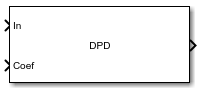# DPD

Digital predistorter

Since R2019a

•Libraries:
Communications Toolbox / RF Impairments Correction

## Description

Apply digital predistortion (DPD) to a complex baseband signal using a memory polynomial to compensate for nonlinearities in a power amplifier. For more information, see Digital Predistortion and Optimizing Estimator Polynomial Degree and Memory Depth.

This icon shows the block with all ports enabled.## Ports

### Input

expand all

Input baseband signal, specified as a column vector. This port is unnamed until the Coefficient source parameter is set to ```Input port```.

Data Types: `double`
Complex Number Support: Yes

Memory-polynomial coefficients, specified as a matrix. The number of rows in the matrix must equal the memory depth of the memory polynomial.

• If the Polynomial type parameter is set to `Memory polynomial`, the number of columns in the matrix is the degree of the memory polynomial.

• If Polynomial type is set to ```Cross-term memory polynomial```, the number of columns in the matrix must equal m(n-1)+1. m is the memory depth of the polynomial, and n is the degree of the memory polynomial.

Example: ```complex([1 0 0 0 0; 0 0 0 0 0; 0 0 0 0 0])```

#### Dependencies

To enable this port, set the Coefficient source parameter to `Input port`.

Data Types: `double`
Complex Number Support: Yes

### Output

expand all

Predistorted baseband signal, returned as a column vector of the same length as the input signal.

## Parameters

expand all

Polynomial type used for predistortion, specified as one of these values:

• `Memory polynomial` — Computes predistortion coefficients by using a memory polynomial without cross terms

• `Cross-term memory polynomial` — Computes predistortion coefficients by using a memory polynomial with cross terms

Source of the memory polynomial coefficients, specified as one of these values:

• `Property` — Specify this value to use the Coefficients parameter to define the memory-polynomial coefficients

• `Input port` — Specify this value to use the Coef input port to define the memory-polynomial coefficients

Memory-polynomial coefficients, specified as a matrix. The number of rows must equal the memory depth of the memory polynomial.

• If the Polynomial type is set to ```Memory polynomial```, the number of columns is the degree of the memory polynomial.

• If the Polynomial type is set to ```Cross-term memory polynomial```, the number of columns must equal m(n-1)+1. m is the memory depth of the polynomial, and n is the degree of the memory polynomial.

#### Dependencies

To enable this parameter, set Coefficient source to `Property`.

Type of simulation to run, specified as `Code generation` or `Interpreted execution`.

• `Code generation` — Simulate the model by using generated C code. The first time you run a simulation, Simulink® generates C code for the block. The model reuses the C code for subsequent simulations unless the model changes. This option requires additional startup time, but the speed of the subsequent simulations is faster than with the `Interpreted execution` option.

• `Interpreted execution` — Simulate the model by using the MATLAB® interpreter. This option shortens startup time, but the speed of subsequent simulations is slower than with the ```Code generation``` option. In this mode, you can debug the source code of the block.

## Block Characteristics

 Data Types `double` | `single` Multidimensional Signals `no` Variable-Size Signals `yes`

## Algorithms

expand all

 Morgan, Dennis R., Zhengxiang Ma, Jaehyeong Kim, Michael G. Zierdt, and John Pastalan. "A Generalized Memory Polynomial Model for Digital Predistortion of Power Amplifiers." IEEE® Transactions on Signal Processing. Vol. 54, Number 10, October 2006, pp. 3852–3860.

 M. Schetzen. The Volterra and Wiener Theories of Nonlinear Systems. New York: Wiley, 1980.